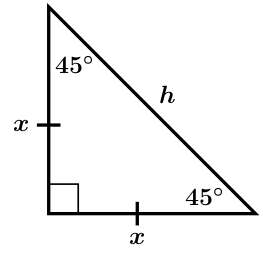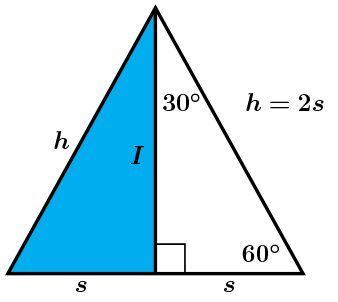# GEOM 2 | Lesson 2 | Watch (Special Right Triangles)# Special Right Triangles

“What did you notice about the triangles?”

This is the 45-45-90 degree Triangle Theorem: “The length of the hypotenuse in a 45-45-90 degree triangle is $$\sqrt{2}$$ times the length of the leg.”

### 45-45-90 Triangles

##### Finding the Length of the Hypotenuse• The Pythagorean Theorem can be used to find the length of the hypotenuse when given the length of the legs.
• $$x^2+x^2=h^2$$
• $$2x^2=h^2$$
• $$\sqrt{x^2*2}=h^2$$
• $$x\sqrt{2}=h$$
• You can save a lot of time and work if you remember $h=x\sqrt{2}$

Prove this theorem using the Pythagorean Theorem to verify the theorem both abstractly, and then using the values from the triangles.

Next, look at the 30-60-90 degree Triangle Theorem: “The length of the hypotenuse in a 30-60-90 degree triangle is 2 times the length of the shortest leg, and the length of the longer leg is $$\sqrt{3}$$ times the length of the shortest leg.”

### 30-60-90 Triangles

##### Understanding the Shortcut for Finding the Length of the Long LegThe Pythagorean Theorem is used to show the relationship between the long side, I, the short side, s, and the hypothenuse, h.

$$s^2+I^2=h^2$$ →  $$I^2=3s^2$$

$$s^2+I^2=(2s^2)^2$$ →  $$\sqrt{I^2}=\sqrt{3s^2}$$

$$I^2=4s^2-s^2$$ → $$I=s\sqrt{3}$$

Prove this theorem using the Pythagorean Theorem to verify the theorem both abstractly, and then using the values from the triangles.

Mathematicians have used these patterns to find the missing angles and sides in what they call “Special Right Triangles”.

Watch this Khan Academy video on Special Right Triangles to see some applications of Special Right Triangles.  Return to this page by using the back arrow.

Special Right Triangle video